### Calculating a Rolling Offset Between Two Pipes

Here is a nice little MEP related external command that I implemented to calculate a rolling offset for pipes at the CASE BIM Hackathon at AU, prompted by a suggestion by Harry Mattison and Matthew Nelson.

It calculates the rolling offset between two selected pipes and generates a model line in the Revit model to display the result.

In brief, a rolling offset is an angled pipe segment that connects two pipes with each other.

For instance, imagine a situation with two parallel horizontal pipes, offset from each other in all three directions, X, Y and Z.

The task consists in calculating the appropriate angled segment to connect the two.

Maybe the best way to explain the task is to show the solution in action before looking at the detailed implementation.

Given the two parallel offset pipes, one needs to decide how far along the axis defined by their common direction to place the angled segment.

I decided to choose the midpoint defined by the two pipes' endpoints that are farthest apart, and place the angled segment so that its midpoint coincides with that.

Here is a 52-second video of the rolling offset add-in recorded live in situ at the CASE BIM Hackathon:

In case you prefer stills, here are six screen snapshots highlighting the situation, three before and three after executing the command: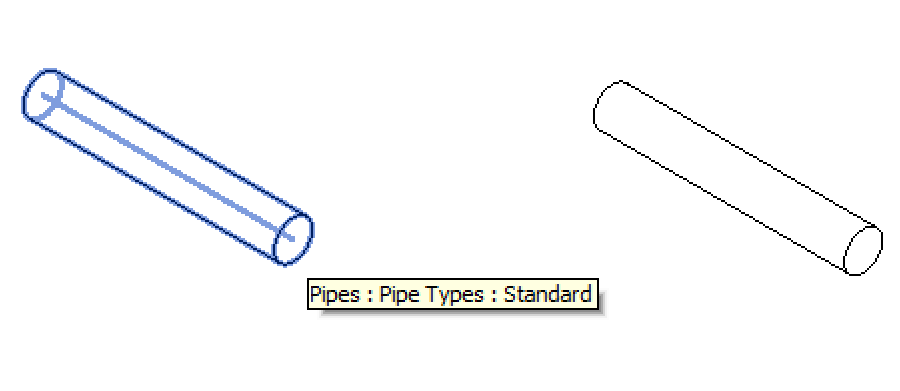3D view before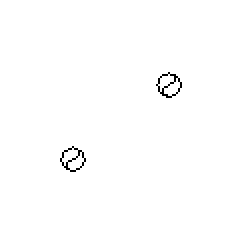East view before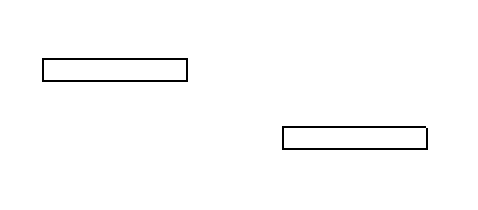North view before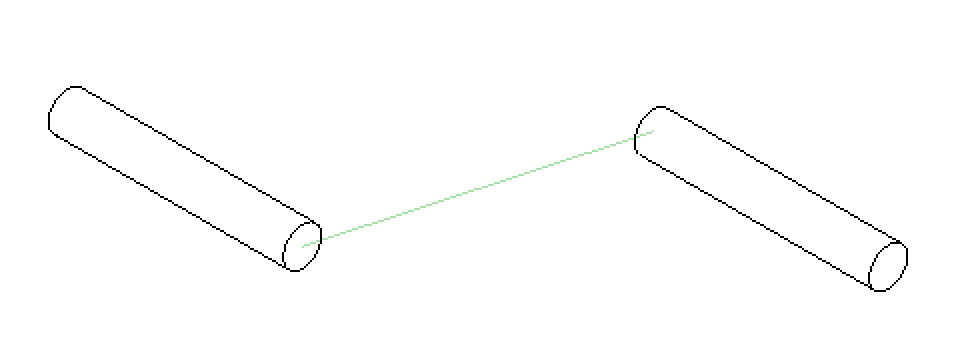3D view after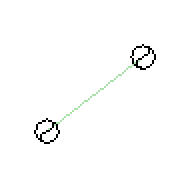East view after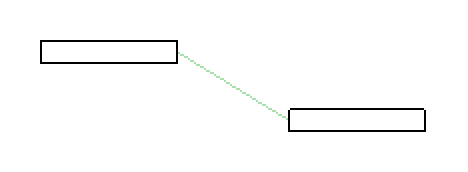North view after

If you look carefully, you will notice that both selected pipes are shortened by the external command. A model line is drawn at a 45-degree angle to the existing pipes to connect the two correspondingly adjusted endpoints.

The algorithm supports any other angle as well, of course, e.g. 30 or 60 degrees; it is currently hard coded.

#### Rolling Offset Calculation Implementation

I implemented the external code calculating the rolling offset and generating a model line to represent the result as a new external command CmdRollingOffset in The Building Coder samples.

For a maximum of flexibility, comfort and efficiency in testing it, it supports three different possibilities for selecting the two pipes:

• Run in a model containing exactly two parallel offset pipe elements, they will be automatically selected.
• Pre-select two pipes before launching the command.
• Post-select them when prompted.

Here is the entire command implementation:

```[Transaction( TransactionMode.Manual )]
class CmdRollingOffset : IExternalCommand
{
const string _prompt
= "Please run this in a model containing "
+ "exactly two parallel offset pipe elements, "
+ "and they will be "
+ "automatically selected. Alternatively, pre-"
+ "select two pipe elements before launching "
+ "this command, or post-select them when "
+ "prompted.";

/// <summary>
/// Allow selection of curve elements only.
/// </summary>
class PipeElementSelectionFilter : ISelectionFilter
{
public bool AllowElement( Element e )
{
return e is Pipe;
}

public bool AllowReference( Reference r, XYZ p )
{
return true;
}
}

public Result Execute(
ExternalCommandData commandData,
ref string message,
ElementSet elements )
{
UIApplication uiapp = commandData.Application;
UIDocument uidoc = uiapp.ActiveUIDocument;
Application app = uiapp.Application;
Document doc = uidoc.Document;

// Select all pipes in the entire model.

List<Pipe> pipes = new List<Pipe>(
new FilteredElementCollector( doc )
.OfClass( typeof( Pipe ) )
.ToElements()
.Cast<Pipe>() );

int n = pipes.Count;

// If there are less than two,
// there is nothing we can do.

if( 2 > n )
{
message = _prompt;
return Result.Failed;
}

// If there are exactly two, pick those.

if( 2 < n )
{
// Else, check for a pre-selection.

pipes.Clear();

Selection sel = uidoc.Selection;

n = sel.Elements.Size;

Debug.Print( "{0} pre-selected elements.",
n );

// If two or more model pipes were pre-
// selected, use the first two encountered.

if( 1 < n )
{
foreach( Element e in sel.Elements )
{
Pipe c = e as Pipe;

if( null != c )
{

if( 2 == pipes.Count )
{
Debug.Print( "Found two model pipes, "
+ "ignoring everything else." );

break;
}
}
}
}

// Else, prompt for an
// interactive post-selection.

if( 2 != pipes.Count )
{
pipes.Clear();

try
{
Reference r = sel.PickObject(
ObjectType.Element,
new PipeElementSelectionFilter(),
"Please pick first pipe." );

pipes.Add( doc.GetElement( r.ElementId )
as Pipe );
}
catch( Autodesk.Revit.Exceptions
.OperationCanceledException )
{
return Result.Cancelled;
}

try
{
Reference r = sel.PickObject(
ObjectType.Element,
new PipeElementSelectionFilter(),
"Please pick second pipe." );

pipes.Add( doc.GetElement( r.ElementId )
as Pipe );
}
catch( Autodesk.Revit.Exceptions
.OperationCanceledException )
{
return Result.Cancelled;
}
}
}

// Extract data from the two selected pipes.

Curve c0 = (pipes.Location as LocationCurve).Curve;
Curve c1 = (pipes.Location as LocationCurve).Curve;

if( !(c0 is Line) || !(c1 is Line) )
{
message = _prompt
+ " Expected straight pipes.";

return Result.Failed;
}

XYZ p00 = c0.GetEndPoint( 0 );
XYZ p01 = c0.GetEndPoint( 1 );

XYZ p10 = c1.GetEndPoint( 0 );
XYZ p11 = c1.GetEndPoint( 1 );

XYZ v0 = p01 - p00;
XYZ v1 = p11 - p10;

if( !Util.IsParallel( v0, v1 ) )
{
message = _prompt
+ " Expected parallel pipes.";

return Result.Failed;
}

// Select the two pipe endpoints that are
// farthest apart.

XYZ p0 = p00.DistanceTo( p10 ) > p01.DistanceTo( p10 )
? p00
: p01;

XYZ p1 = p10.DistanceTo( p0 ) > p11.DistanceTo( p0 )
? p10
: p11;

XYZ pm = 0.5 * ( p0 + p1 );

XYZ v = p1 - p0;

if( Util.IsParallel( v, v0 ) )
{
message = "The selected pipes are colinear.";
return Result.Failed;
}

XYZ z = v.CrossProduct( v1 );
XYZ w = z.CrossProduct( v1 ).Normalize();

// Offset distance perpendicular to pipe direction

double distanceAcross = Math.Abs(
v.DotProduct( w ) );

// Distance between endpoints parallel
// to pipe direction

double distanceAlong = Math.Abs(
v.DotProduct( v1.Normalize() ) );

Debug.Assert( Util.IsEqual( v.GetLength(),
Math.Sqrt( distanceAcross * distanceAcross
+ distanceAlong * distanceAlong ) ),
"expected Pythagorean equality here" );

// The required offset pipe angle.

double angle = 45 * Math.PI / 180.0;

// The angle on the other side.

double angle2 = 0.5 * Math.PI - angle;

double length = distanceAcross * Math.Tan( angle2 );

double halfLength = 0.5 * length;

// How long should the pipe stubs become?

double remainingPipeLength
= 0.5 * (distanceAlong - length);

if( 0 > v1.DotProduct( v ) )
{
v1.Negate();
}

v1 = v1.Normalize();

XYZ q0 = p0 + remainingPipeLength * v1;

XYZ q1 = p1 - remainingPipeLength * v1;

using( Transaction tx = new Transaction( doc ) )
{
tx.Start( "Rolling Offset" );

// Trim or extend existing pipes

(pipes.Location as LocationCurve).Curve
= Line.CreateBound( p0, q0 );

(pipes.Location as LocationCurve).Curve
= Line.CreateBound( p1, q1 );

// Add a model line for the rolling offset pipe

Creator creator = new Creator( doc );

Line line = Line.CreateBound( q0, q1 );

creator.CreateModelCurve( line );

tx.Commit();
}
return Result.Succeeded;
}
}
```

I hope you find this interesting and useful.

Here are two comments I received on this from Harry and Matt:

• I'm pretty sure this is the most bad-ass thing anyone did at the Hackathon. Nice work Jeremy!
• I second that! I wasn't there but I will pretend I was!

The code presented above is available from The Building Coder samples GitHub repository, and the version discussed here is release 2014.0.106.1.

Tere hommikust!